﻿ 暗物质效应的多重物理机制研究

# 暗物质效应的多重物理机制研究On Multiple Mechanisms of Dark Matter Effect

Abstract: Mass Complex Space Theory (MCST) holds that basic particles are the local representation of the interaction of complex space of matter, gravitational field is the body of space, and pure empty space does not exist. MCST attributes the mechanism of gravitational action to the exchange of gravitational field flow between objects, and then infers that dark matter effect originates fromthe secondary distribution of gravitational field flow. Further study shows that the exchange of gravitational field flow between objects only plays a structural secondary role in dark matter effect. By introducing the generalized centripetal force formula, we can further infer that dark matter effect is also due to the increasing velocity of object with the same kinetic energy in lower vacuum density environment. Finally, through the study of vortex effect of gravitational field flow, we can conclude that the free motion of celestial body caused by circulation of gravitational field plays a critical role in dark matter effect.

1. 研究背景

2. 物质复空间理论简介

MCST第一公设：物质是由对立统一的虚实空间相互作用生成。

MCST第二公设：实空间是收敛的引力场，虚空间是发散的斥力场。

MCST第三公设：物质是超循环物质态的局域耦合与分化的表象。

MCST第四公设：超循环物质态的运动遵循物极必反的自然法则。

MCST第五公设：实空间是物质的虚态，虚空间是物质的实态。

MCST第六公设：多质体引力场流的分布遵守最小作用原理。

MCST第七公设：质体的运动是其引力场空间外在不平衡的效应。

MCST第八公设：在物质复空间的临界域，引力场流速等于引力场波速。

MCST第九公设：物质复空间场流的分布具有力学惯性。

MCST第十公设：质体本征复空间的临界域截面与其本征质量成正比。

MCST第一定理：宇宙的极大尺寸与极小尺度是对立统一。

MCST第二定理：相互靠近的一对粒子，二者复空间的临界域均增大。

MCST第三定理：距离其它质体足够远的一对质体，各自截获对方的引力质量与二者的本征质量成反比。

MCST第四定理：质体从背景真空中截获的引力质量与质体的本征质量成正比。

MCST第五定理：质体的引力质量恒等于其惯性质量。

MCST第六定理：对于近距离作用的一对质体，质体在实空间截获的引力质量，在虚空间全部返还给与其作用的质体。

MCST第七定理：稳态的天体系统，中心天体的引力作用质量总是小于其本征质量的，外围天体的引力作用质量总是大于其本征质量的。

MCST第二公设的补充说明：由于实空间是负能态的，本公设中所谓的收敛与发散均是正能化的，正能量的收敛等价于负能量的发散(可类比电子运动方向和电流方向相反)。因此，负能态的引力场实为发散的负能量场。

3. 基于惯性系的物理学研究

19世纪的德国物理学家朗奇提出从物理概念的基础寻找消除绝对空间概念的方法。这个方法是用惯性系取代绝对空间，将牛顿力学体系建立在惯性系的基础上。事实上，牛顿力学和狭义相对论都是建立惯性系基础之上的“惯性系物理学”。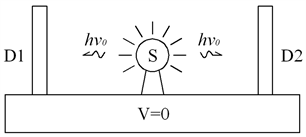Figure 1. Assumed stationary inertial frame S in absolute space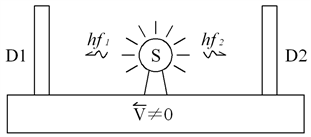Figure 2. Assumed motioning inertial frame S in absolute space

${f}_{1}=\sqrt{\frac{C+V}{C-V}}{v}_{0}$ (1)

${f}_{2}=\sqrt{\frac{C-V}{C+V}}{v}_{0}$ (2)

${{f}^{\prime }}_{1}=\sqrt{\frac{C-V}{C+V}}v$ (3)

${{f}^{\prime }}_{2}=\sqrt{\frac{C+V\text{​}}{C-V}}v$ (4)

${v}_{1}=\sqrt{\frac{C-V}{C+V}}{f}_{1}={v}_{0}$ (5)

${v}_{2}=\sqrt{\frac{C+V}{C-V}}{f}_{2}={v}_{0}$ (6)

4. 质体的运动活力

MCST第八定理：动能相等且本征质量相同的质体的运动速度，与其所处的真空背景密度成反比。

$\frac{{V}_{1}}{{V}_{2}}=\frac{{C}_{1}}{{C}_{2}}=\frac{{H}_{2}}{{H}_{1}}$

$\frac{{V}_{1}}{{C}_{1}}=\frac{{V}_{2}}{{C}_{2}}$

MCST第八定理表明：在任何惯性系内，我们都不能通过测量不同动体的速度的方式去测出光速的变化。因此，狭义相对论的“相对性原理”在物质复空间理论中仍然是有效的。当我们选择以时间为基准时，物质复空间理论对广义相对论也是兼容的。

$F=m{\left(v/\eta \right)}^{2}/r=\frac{m{v}^{2}}{r}/{\eta }^{2}=\frac{m{v}^{2}}{r}/{\left(\frac{{C}_{m}}{{C}_{E}}\right)}^{2}=\frac{m{v}^{2}}{r}/{\left(\frac{\Sigma |{g}_{Ei}|}{\Sigma |{g}_{mi}|}\right)}^{2}=\frac{m{v}^{2}{\left(\Sigma |{g}_{mi}|\right)}^{2}}{r{\left(\Sigma |{g}_{Ei}|\right)}^{2}}$

$\eta$ 为质体的运动活力系数，引力场的标量叠加决定真空磁导率，真空磁导率决定真空光速，真空光速决定质体的最大运动速度和运动活力。 $\sum |{g}_{m}{}_{i}|$ 为质体m的背景引力场标量叠加(包括m自身的引力场)； $\sum |{g}_{E}{}_{i}|$ 为地球的背景引力场标量叠加(包括地球自身的引力场)。

5. 宇宙空间平均质能的计算方法

$N=\frac{4\pi {R}^{3}-4\pi {\left(R-1\right)}^{3}}{3{m}^{3}}$

$\sum |{g}_{R}|=\frac{G\left({E}_{0}\frac{4\pi {R}^{3}-4\pi {\left(R-1\right)}^{3}}{3{m}^{3}}\right)/{C}^{2}}{{R}^{2}}=\frac{4\pi G{E}_{0}\left[{R}^{3}-{\left(R-1\right)}^{3}\right]}{3{R}^{2}{C}^{2}{m}^{3}}=\frac{4\pi G{E}_{0}\left[1-1/R+1/3{R}^{2}\right]}{{C}^{2}{m}^{3}}$

P点的相对真空密度等于宇宙空间的平均质能在P点引力场的标量叠加：

$\underset{R=1}{\overset{lU}{\sum }}\sum |{g}_{R}|=\underset{R=1}{\overset{lU}{\sum }}\frac{4\pi G{E}_{0}\left[1-1/R+1/3{R}^{2}\right]}{{C}^{2}{m}^{3}}=\frac{4\pi {G}_{0}{E}_{0}}{{C}^{2}{m}^{3}}\left[lU-\left(\mathrm{ln}lU+0.57722\right)+{\pi }^{2}/18\right]\approx \frac{4\pi G{E}_{0}lU}{{C}^{2}{m}^{3}}$

$\begin{array}{l}\frac{4\pi G{E}_{0}lU}{{C}^{2}{m}^{3}}={H}_{0}\\ {E}_{0}=\frac{{C}^{2}{H}_{0}{m}^{3}}{4\pi GlU}=\frac{{C}^{2}{H}_{0}lPm}{4\pi G}=1.7324×{10}^{-9}{H}_{0}\text{\hspace{0.17em}}\text{N}\cdot {\text{s}}^{2}\end{array}$

6. 天体在涡旋引力场中的运动规律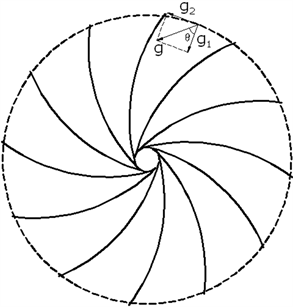Figure 3. Analysis of vortex gravitational field

$\frac{GM}{{R}^{2}}\mathrm{cos}\theta =\frac{{\left(V-{V}_{0}\right)}^{2}}{R}$Figure 4. Entity model of movement of vortex fluid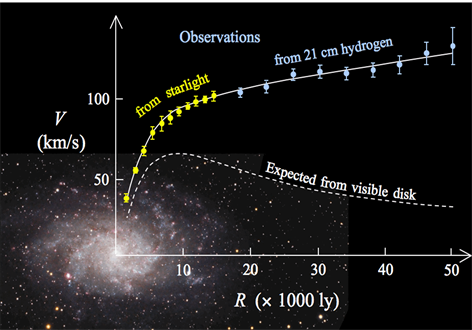Figure 5. Big deviation of expected value and observed value of matter in galaxy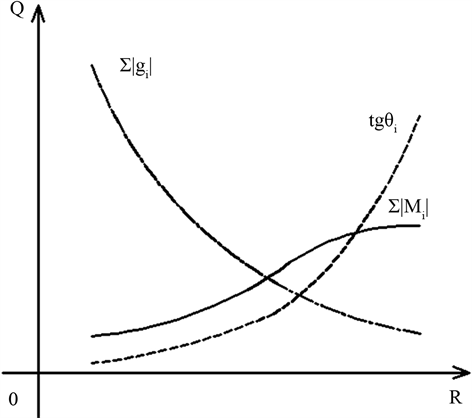Figure 6. Typical distribution of matter and the field effects in galaxy

7. 物质复空间理论与东西方主流宇宙观的对照Table 1. Comparisons between MCST and the mainstream world views of the east and the west

8. 总结

9. 展望——基于MCST整合量子力学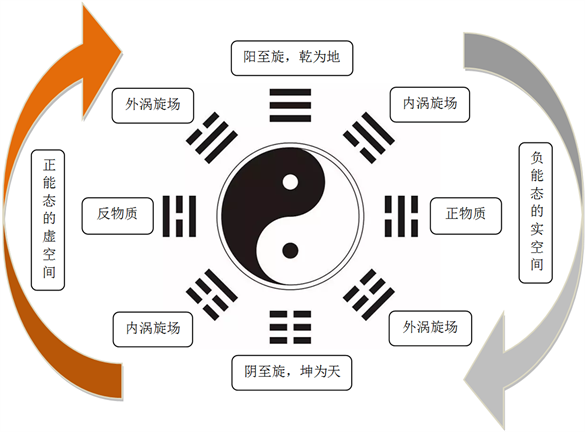Figure 7. Panorama of the movement of mass complex space

 武沧海. 物质复空间理论[J]. 现代物理, 2019, 9(3): 130-148.

 汤川秀树. 创造力与直觉[M]. 河北: 河北科学技术出版社, 2000: 142.

 爱因斯坦. 狭义与广义相对论浅说[M]. 上海: 上海科学技术出版社, 1964.

 爱因斯坦. 狭义与广义相对论浅说[M]. 北京: 北京大学出版社, 2006: 177.

 阎康年. 牛顿的科学发现与科学思想[M]. 长沙: 湖南教育出版社, 1989: 378.

 https://www.toutiao.com/i6280810380956533250

Top